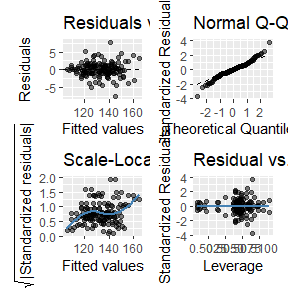# Diagnostic Plots

#### Running Example Requires gglm (White 2023)

#Install gglm
install.packages("gglm")
require(gglm)

## Plotting Model Diagnostics

The code below demonstrates how to plot model diagnostics for rmcorr. There are four diagnostic plots assessing:
1. Residuals vs. Fitted values: Linearity
2. Quantile-Quantile (Q-Q): Normality of residuals
3. Scale-Location: Equality of variance (homoscedasticity)
4. Residuals vs. Leverage: Influential observations

raz.rmc <- rmcorr(participant = Participant, measure1 = Age,
measure2 = Volume, dataset = raz2005)
#> Warning in rmcorr(participant = Participant, measure1 = Age, measure2 = Volume,
#> : 'Participant' coerced into a factor

#Using gglm
gglm(raz.rmc$model)#using base R #plot(raz.rmc$model)

How much do violations of these assumptions matter? It depends. General Linear Model (GLM) is typically robust to deviations from the above assumptions, but severe violations may produce misleading results (Gelman, Hill, and Vehtari 2020). Also, the reason(s) for violations can matter: “Violations of assumptions may result from problems in the dataset, the use of an incorrect regression model, or both” (Cohen et al. 2013, 117).

Cohen, Jacob, Patricia Cohen, Stephen G West, and Leona S Aiken. 2013. Applied Multiple Regression/Correlation Analysis for the Behavioral Sciences. Routledge.
Gelman, Andrew, Jennifer Hill, and Aki Vehtari. 2020. Regression and Other Stories. Cambridge University Press.
White, Grayson. 2023. Gglm: Grammar of Graphics for Linear Model Diagnostic Plots. https://CRAN.R-project.org/package=gglm.